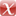# A Submatrix-Based Method for Approximate Matrix Function Evaluation in the Quantum Chemistry Code CP2K

M. Lass, R. Schade, T. Kühne, C. Plessl, (2020).

Preprint | English
Abstract
Electronic structure calculations based on density-functional theory (DFT) represent a significant part of today's HPC workloads and pose high demands on high-performance computing resources. To perform these quantum-mechanical DFT calculations on complex large-scale systems, so-called linear scaling methods instead of conventional cubic scaling methods are required. In this work, we take up the idea of the submatrix method and apply it to the DFT computations in the software package CP2K. For that purpose, we transform the underlying numeric operations on distributed, large, sparse matrices into computations on local, much smaller and nearly dense matrices. This allows us to exploit the full floating-point performance of modern CPUs and to make use of dedicated accelerator hardware, where performance has been limited by memory bandwidth before. We demonstrate both functionality and performance of our implementation and show how it can be accelerated with GPUs and FPGAs.
Publishing Year
LibreCat-ID

### Cite this

Lass M, Schade R, Kühne T, Plessl C. A Submatrix-Based Method for Approximate Matrix Function Evaluation in the Quantum Chemistry Code CP2K. 2020.
Lass, M., Schade, R., Kühne, T., & Plessl, C. (2020). A Submatrix-Based Method for Approximate Matrix Function Evaluation in the Quantum Chemistry Code CP2K.
@article{Lass_Schade_Kühne_Plessl_2020, title={A Submatrix-Based Method for Approximate Matrix Function Evaluation in the Quantum Chemistry Code CP2K}, author={Lass, Michael and Schade, Robert and Kühne, Thomas and Plessl, Christian}, year={2020} }
Lass, Michael, Robert Schade, Thomas Kühne, and Christian Plessl. “A Submatrix-Based Method for Approximate Matrix Function Evaluation in the Quantum Chemistry Code CP2K,” 2020.
M. Lass, R. Schade, T. Kühne, and C. Plessl, “A Submatrix-Based Method for Approximate Matrix Function Evaluation in the Quantum Chemistry Code CP2K.” 2020.
Lass, Michael, et al. A Submatrix-Based Method for Approximate Matrix Function Evaluation in the Quantum Chemistry Code CP2K. 2020.

### Export

Marked Publications

Open Data LibreCat

### SourcesarXiv 2004.10811